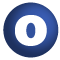# NoPosition

#### object NoPosition extends Position

Undefined position

1. Position
2. AnyRef
3. Any

### Value Members

1. #### def <(that: Position): Boolean

Compare this position to another, by first comparing their line numbers, and then -- if necessary -- using the columns to break a tie

Compare this position to another, by first comparing their line numbers, and then -- if necessary -- using the columns to break a tie.

definition classes: Position
2. #### def column: Int

The column number referred to by the position; column numbers start at 1

The column number referred to by the position; column numbers start at 1

definition classes: NoPositionPosition
3. #### def equals(arg0: Any): Boolean

This method is used to compare the receiver object (`this`) with the argument object (`arg0`) for equivalence

This method is used to compare the receiver object (`this`) with the argument object (`arg0`) for equivalence.

The default implementations of this method is an equivalence relation:

• It is reflexive: for any instance `x` of type `Any`, `x.equals(x)` should return `true`.
• It is symmetric: for any instances `x` and `y` of type `Any`, `x.equals(y)` should return `true` if and only if `y.equals(x)` returns `true`.
• It is transitive: for any instances `x`, `y`, and `z` of type `AnyRef` if `x.equals(y)` returns `true` and `y.equals(z)` returns `true`, then `x.equals(z)` should return `true`.

If you override this method, you should verify that your implementation remains an equivalence relation. Additionally, when overriding this method it is often necessary to override `hashCode` to ensure that objects that are "equal" (`o1.equals(o2)` returns `true`) hash to the same `Int` (`o1.hashCode.equals(o2.hashCode)`).

arg0

the object to compare against this object for equality.

returns

`true` if the receiver object is equivalent to the argument; `false` otherwise.

definition classes: AnyRef ⇐ Any
4. #### def hashCode(): Int

Returns a hash code value for the object

Returns a hash code value for the object.

The default hashing algorithm is platform dependent.

Note that it is allowed for two objects to have identical hash codes (`o1.hashCode.equals(o2.hashCode)`) yet not be equal (`o1.equals(o2)` returns `false`). A degenerate implementation could always return `0`. However, it is required that if two objects are equal (`o1.equals(o2)` returns `true`) that they have identical hash codes (`o1.hashCode.equals(o2.hashCode)`). Therefore, when overriding this method, be sure to verify that the behavior is consistent with the `equals` method.

definition classes: AnyRef ⇐ Any
5. #### def line: Int

The line number referred to by the position; line numbers start at 1

The line number referred to by the position; line numbers start at 1

definition classes: NoPositionPosition
6. #### def lineContents: String

The contents of the line numbered `lnum' (must not contain a new-line character)`

The contents of the line numbered ```lnum' (must not contain a new-line character). ```

definition classes: NoPositionPosition
7. #### def longString: String

Returns a more visual representation of this position

Returns a more visual representation of this position. More precisely, the resulting string consists of two lines:

1. the line in the document referred to by this position
2. a caret indicating the column

Example:

```    List(this, is, a, line, from, the, document)
```

definition classes: NoPositionPosition
8. #### def toString(): String

Returns a string representation of the `Position', of the form `line

Returns a string representation of the `Position', of the form `line.column' @return a string representation of the object.

*/

definition classes: NoPositionPosition ⇐ AnyRef ⇐ Any